Courses

# Test: RD Sharma: Real Numbers- 2

## 25 Questions MCQ Test Mathematics (Maths) Class 10 | Test: RD Sharma: Real Numbers- 2

Description
This mock test of Test: RD Sharma: Real Numbers- 2 for Class 10 helps you for every Class 10 entrance exam. This contains 25 Multiple Choice Questions for Class 10 Test: RD Sharma: Real Numbers- 2 (mcq) to study with solutions a complete question bank. The solved questions answers in this Test: RD Sharma: Real Numbers- 2 quiz give you a good mix of easy questions and tough questions. Class 10 students definitely take this Test: RD Sharma: Real Numbers- 2 exercise for a better result in the exam. You can find other Test: RD Sharma: Real Numbers- 2 extra questions, long questions & short questions for Class 10 on EduRev as well by searching above.
QUESTION: 1

### The HCF of two consecutive numbers is

Solution:

The HCF of two consecutive numbers is always 1.

QUESTION: 2

### Find the largest number which divides 62,132,237 to leave the same reminder

Solution:

Trick is HCF of (237-132), (132-62), (237-62)
= HCF of (70,105,175) = 35

QUESTION: 3

### The product of two numbers is -20/9. If one of the numbers is 4, find the other.

Solution:

We have two numbers such that their product is equal to -20/9.
So we have x*y=-20/9
One no. is given 4, so
x*4=-20/9
x=-20/9 = 4
x=-20/9 x 1/4=-5/9

QUESTION: 4

All non-terminating and non-recurring decimal numbers are

Solution:

All non-terminating and non-recurring decimal numbers are irrational numbers.

QUESTION: 5

The decimal expansion of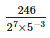terminates after:

Solution: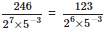Therefore,will terminate after 6 places of decimals.

QUESTION: 6

If 112 = q×6+r, then the possible values of r are:

Solution:

For the relation x = qy+r, 0 ⩽ r < y So, here r lies between 0 ⩽ r < 6. Hence r = 0, 1, 2, 3, 4, 5

QUESTION: 7

The HCF of two consecutive even numbers is

Solution:

The HCF of two consecutive even numbers is 2.

QUESTION: 8

The largest number of 4 digits exactly divisible by 12, 15, 18 and 27 is

Solution:

LCM (12, 15, 18, 27) = 540
Now, largest four digit number = 9999
∴ 9999 ÷ 540 = 18 x 540 + 279 (Remainder = 279) Therefore, the largest number of 4 digits exactly divisible by 12, 15, 18 and 27 is 9999 – 279 = 9720

QUESTION: 9

Which of the following is a rational number?

Solution:

√9 is a rational number because √9 = 3 and 3 is a rational number.

QUESTION: 10

A rational number can be expressed as a terminating decimal if the denominator has the factors

Solution:

A rational number can be expressed as a terminating decimal if the denominator has the factors 2 or 5 only.

QUESTION: 11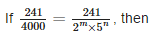Solution: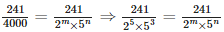Comparing the denominators of both fractions, we have m = 5 and n = 3

QUESTION: 12

By Euclid’ division lemma x = qy + r,x > y,, the value of q and r for x = 27 and y = 5 are:

Solution:

x = qy+r ⇒  27= 5×5+2 ⇒ q = 5,r = 2

QUESTION: 13

The LCM of two consecutive numbers is

Solution:

The LCM of two consecutive numbers is their product always.

QUESTION: 14

Pairs of natural numbers whose least common multiple is 78 and the greatest common divisor is 13 are:

Solution:

Let the no.s be a & b,

GCD [ a , b ] = 13;
=> Let a = 13m and b = 13n

Now, LCM [ a , b ] = 78
=> 13m | 78
=> m | 6 and similarly, n | 6; --> Also, m does not divide n

.'. Possible values of (m,n) are :->
[ 1 , 6 ] , [ 2 , 3 ]
.'. Possible values for ( a , b ) are :-> [ 13 , 78 ] , [ 26 , 39 ]

QUESTION: 15

If ‘p’ is a prime number, then √p is

Solution:

√p is an irrational number because square root of every prime number is an irrational number.

QUESTION: 16

A rational number can be expressed as a non-terminating repeating decimal if the denominator has the factors

Solution:

A rational number can be expressed as a non-terminating repeating decimal if the denominator has the factors other than 2 or 5.

QUESTION: 17

If 9x+2 = 240+9x, then the value of ‘x’ is

Solution: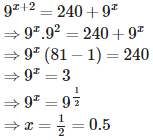QUESTION: 18

If A = 2n + 13, B = n + 7, where n is a natural number then HCF of A and B is:

Solution:

A = 2n + 13
B = n + 7

1) If the value of n = 1, then

A = (2*1) + 13
= 2 + 13
= 15

B = 1 + 7
= 8

Now, A = 15 and B = 8
HCF of 15 and 8 is 1.

2) If the value of n =2

A = (2*2) + 13
= 4 + 13
= 17

B = 2 + 7
= 9

A = 17 and B = 9
HCF of 17 and 9 is 1.

3) If the value of n 3

A = (2*3) + 13
= 6 + 13
= 19

B = 3 + 7
= 10

A = 19 and B = 10
HCF of 19 and 10 is 1

4) If the value of n = 4
A = (2*4) + 13
= 8 +13
= 21

B = 4 + 7
= 11

A = 21 and B = 11

HCF of 21 and 11 is 1

5) If the value of n = 5
A = (2*5) + 13
= 10 + 13
= 23

B = 5 + 7
= 12

A = 23 and B = 12
HCF of 23 and 12 is 1

6) If the value of n = 6
A = (2*6) + 13
= 12 + 13
= 25

B = 6 + 7
= 13

A = 25 and B = 13
HCF of 25 and 13 is 1

7) If the value of n = 7
A = (2*7) + 13
14 + 13
= 27

B = 7 + 7
= 14

A = 27 and B = 14
HCF of 27 and 14 is 1

8) If the value of n = 8
A = (2*8) + 13
16 + 13
= 29

B = 8 + 7
= 15

A = 29 and B = 15
HCF of 29 and 15 is 1

9) If the value of n = 9
A = (2*9) + 13
18 + 13
= 31

B = 9 + 7
= 16

A = 31 and B = 16
HCF of 31 and 16 is 1

10) If the value of n = 10
A = (2*10) + 13
20 + 13
= 33

B = 10 + 7
= 17

A = 33 and B = 17
HCF of 33 and 17 is 1

We have seen that whatever the value n has, the HCF of A and B (separate values they hold in each situation) is always 1. It means we can take any value of n and the HCF will be 1.

QUESTION: 19

The LCM of 24, 60 and 150 is

Solution: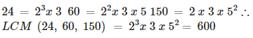QUESTION: 20

The LCM of two number is 45 times their HCF. If one of the numbers is 125 and the sum of HCF and LCM is 1150, the other number is:

Solution:

LCM = 45 HCF
LCM + HCF = 1150
45*HCF + HCF = 1150
46 *HCF = 1150
HCF = 25
Then,
LCM = 45 *25 = 1125.
Now, use the formula,
1st number * second Number = LCM * HCF
125 * second number = 1125 *25
Second Number = 225.

QUESTION: 21

The difference of a rational and an irrational number is always

Solution:

The difference of a rational and an irrational number is always an irrational number.

QUESTION: 22

Which of the following numbers has terminating decimal expansion?

Solution:

3/5 has terminal decimal expansion because terminal decimal expansion should have the denominator 2 or 5 only.

QUESTION: 23

The multiplicative inverse of zero is

Solution:

Some rules associated with Multiplicative Inverse are discussed in following ways :-
Rule 1 = If product of two Fractional Numbers is equal to 1, then each of the Fractional Numbers is the Multiplicative Inverse of other.
Rule 2 = If the product of a Fractional Number and a Whole Number is equal to 1, then each is the Multiplicative Inverse of other.
Rule 3 = Multiplicative Inverse of 1 is also 1.
Rule 4 = Multiplicative Inverse of 0 (zero) does not exists

QUESTION: 24

Any ____________ is of the form 4q + 1 or 4q + 3 for some integer ‘q’.

Solution:

Let a be a given positive odd integer.
Applying Euclid’s Division Lemma to aa and b = 4,,
We have, a = 4q+r where 0 ⩽ r < 4 ⇒ r = 0, 1, 2, 3 ⇒ a = 4q or 4q+1 or 4q+2 or 4q+3
But a = 4q and 4q+2 = 2(2q+1)are clearly even.
Also a = 4q, 4q+1, 4q+2, 4q+3 are consecutive integers, therefore any positive odd integer is of the form 4q+1 and 4q+3 where q is some integer.

QUESTION: 25

The relationship between HCF and LCM of two natural numbers is

Solution:

The product of LCM and HCF of any two given natural numbers is equivalent to the product of the given numbers.

LCM × HCF = Product of the Numbers

Suppose A and B are two numbers, then.

LCM (A & B) × HCF (A & B) = A × B

Example: Prove that: LCM (9 & 12) × HCF (9 & 12) = Product of 9 and 12.
->LCM and HCF of 9 and 12:
9 = 3 × 3 = 3²
12 = 2 × 2 × 3 = 2² × 3
LCM of 9 and 12 = 2² × 3² = 4 × 9 = 36
HCF of 9 and 12 = 3
LCM (9 & 12) × HCF (9 & 12) = 36 × 3 = 108
Product of 9 and 12 = 9 × 12 = 108
Hence, LCM (9 & 12) × HCF (9 & 12) = 108 = 9 × 12